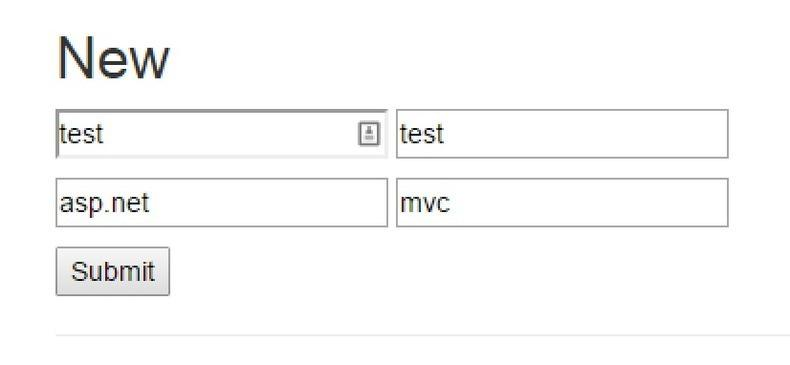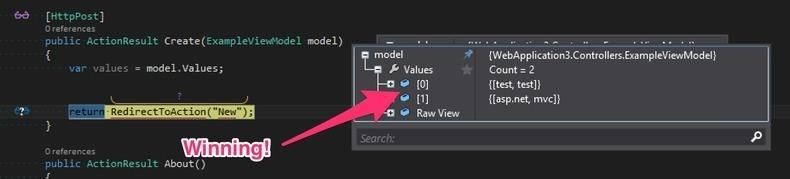When building a line of business application, requests for saving dynamic key/value pairs are inevitable. The “best” way to store dynamic data is in a `Dictionary`, the mother of all flexible data structures. This post will show you the minimum required to post a dictionary to your ASP.NET MVC action so you can meet your business requirements.

The first thing we will need is a view model. For this post, we will use the following model.

``````public class ExampleViewModel
{
public ExampleViewModel()
{
Values = new Dictionary<string, string>();
}

public Dictionary<string,string> Values { get; set; }

public ExampleViewModel Add(string key, string value)
{
return this;
}
}
``````

A simple model with one `Dictionary` property.

Before we get to Post a form, we need to display it. Let’s look at the first Action we will need.

``````public ActionResult New()
{
var model = new ExampleViewModel()

return View(model);
}
``````

We are adding an initial value of test/test. Next, let’s see what the view looks like. This is the most important part of the equation.

``````@model WebApplication3.Controllers.ExampleViewModel @{ ViewBag.Title = "New";
var first = Guid.NewGuid(); var second = Guid.NewGuid(); }

<h2>New</h2>

@using (Html.BeginForm(new { action = "create", controller = "home" })) {
foreach (var kvp in Model.Values) {
<p>
<input type="text" name="Model.Values[@first].Key" value="@kvp.Key" />
<input type="text" name="Model.Values[@first].Value" value="@kvp.Value" />
<input type="hidden" name="Model.Values.Index" value="@first" />
</p>
}

<p>
<input type="text" name="Model.Values[@second].Key" value="" />
<input type="text" name="Model.Values[@second].Value" value="" />
<input type="hidden" name="Model.Values.Index" value="@second" />
</p>

<p>
<input type="submit" value="Submit" />
</p>
}
``````

There are three parts to this view that are important to keep in mind:

1. the `name` property matches our model.
2. the indexer between the brackets doesn’t matter, as long as it is unique.
3. The index value must be passed along as a `hidden` input.

Now, we post our form with the values we want.Once posted, our values show up when debugging.As you can see in the screenshot above, we are seeing our values set correctly.

Once you understand how ASP.NET MVC performs model binding, you can tweak your forms to match those requirements and make it easier to provide value. I hope you found this post informative and helpful.